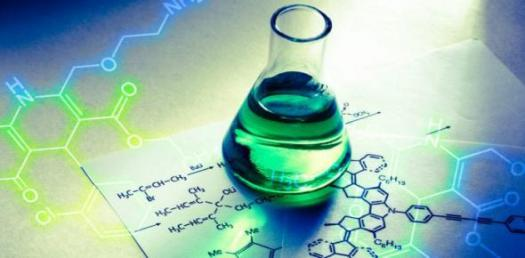# Balancing Chemical Equations! Trivia Questions Quiz

10 Questions | Total Attempts: 112SettingsChemical equations include chemical formulae that represent all substances that are involved in the reaction. When it comes to balancing chemical equations, proper attention must be given to both reactants and product sides of the chemical equation. The rule of balancing the equation is making sure that the number of moles on both sides is equal. Do you know how to balance chemical equations?

• 1.
What law is most important in balancing chemical equations?
• A.

• B.

Charle's law

• C.

Gay Lussac's law

• D.

Law of conservation of mass

• 2.
How many are the standard methods to balancing chemical equations?
• A.

Two methods

• B.

Four methods

• C.

Six methods

• D.

Three methods

• 3.
Which of these is not a method of balancing chemical equations?
• A.

Inspection method

• B.

Matrix method

• C.

Linear equation method

• D.

Elimination method

• 4.
When was the first time a chemical equation was diagrammed?
• A.

1615

• B.

1781

• C.

1923

• D.

1613

• 5.
Who developed the first diagram for a chemical equation?
• A.

Jean Lamarck

• B.

Fred Logan

• C.

Eddie Strong

• D.

Jean Benguin

• 6.
Which of these is irrelevant in chemical equation?
• A.

Forward reaction

• B.

Reactants

• C.

Backward reaction

• D.

Heat of reaction

• 7.
What is the coefficient of a chemical formula without coefficient?
• A.

• B.

• C.

1

• D.

• 8.
What is usually the number of charge of a sodium ion?
• A.

1

• B.

-1

• C.

• D.

-2

• 9.
What is the number of charge of an element in a free state?
• A.

• B.

• C.

3

• D.

0

• 10.
What gas is produced when hydrochloric acid and sodium reacts?
• A.

Chlorine

• B.

Hydrogen

• C.

Sulphur

• D.

Sodium

Related Topics